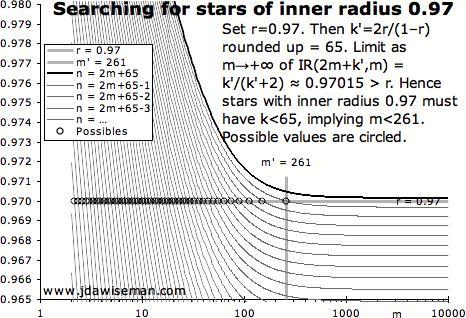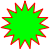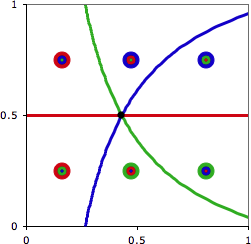# Open Mathematical Questions at jdawiseman.com

Julian D. A. Wiseman, January 2009

Publication history: only at jdawiseman.com/papers/easymath/open_questions.html. Usual disclaimer and copyright terms apply.

Scattered around jdawiseman.com, lodged within other essays, are various unsolved mathematical questions. These might be within the reach of a competent undergraduate student of mathematics. A solution to the first of the three would certainly be worthy of a paper in a mathematical journal, and solutions to either of the others might be worthy of mention.

This list might grow over time.

Those giving serious attention to more than none of these problems are invited to tell this to the author, so that others may be forewarned that an answer is growing. Unless requested otherwise, problems will be marked with the names of those working on them.

## The Chow & Robbins problem: estimating sn/n

This problem taken from The expected value of sn/n ≈ 0.79295350640….

A game is played by repeatedly tossing a fair coin, stopping any time after the first toss. On stopping the score is the proportion of tosses that are heads. Given optimal strategy, what is the expected score?

Assume that there have already been h and t tails, at which state the expected value of the game is defined to be EV(h,t). An approximation to EV(h,t) with h and t both large would allow far more accurate estimation of EV(0,0).

This approximation to EV(h,t) might be the solution to a continuous version of the game. Possible properties of such a continuous game are to be found under “Next Steps” in the linked paper.

Any help with such an approximation would be welcomed.

Relatedly, the data in jdawiseman.com/papers/easymath/coin_all_scores_no_estimated_limits.txt contains all of the computed approximations to the game values. From which I had been calculating an estimated limit using various threes of these rows, with max_t in geometic progression. But using all the data simultaneously should be more robust. Effectively this would fitting a curve of the form limit – α×eβ×max_t, giving lesser weight to the data with smaller max_t. Expert help eagerly sought, probably from a statistician.## Matching stars

This problem taken from The Inner Radius of n/m Stars, in Surds.

Let n and m be integers, with 2 ≤ m < n/2. Consider the bounding polygon of a n/m star (that is, a star with n points each of which connects to the two points m away) inscribed in the unit circle. Such a bounding polygon has 2n points, n on the unit circle and n on the circle of radius Cos[π m / n] / Cos[π (m–1) / n]. Call this expression the “inner radius” of the n/m star.

We wish to characterise the different stars with the same inner radius. And for integer i ≥ 2, stars (6i–2)/i and (18i–6)/(6i–2) have the same inner radius, as do (6i–4)/i and (18i–12)/(6i–3). For example, see the PDFs of overlapping 8/2 and 24/9 stars which have inner radius √(2–√2), and of 10/2 and 30/10 stars with inner radius √((5+√5)/10), and of 14/3 and 42/15 stars.

But are all pairs of stars with equal inner radii from one of these two series? Proof or counter-example welcomed. It is known that any counter-example must have inner radius > 0.998122. (So perhaps the reader will allow a theorem: the only pairs of n/m stars that look like stars and have the same inner radii are from one of these two series.)

The proof that these two series do match is elementary, given the identity Cos[θ] Cos[φ] = ½Cos[θ+φ] + ½Cos[θ–φ].

 (6i–2)/i and (18i–6)/(6i–2) stars match ⇔ 0 = Cos[π i/(6i–2)] / Cos[π (i–1)/(6i–2)] – Cos[π (6i–2)/(18i–6)] / Cos[π (6i–3)/(18i–6)] ⇔ 0 = Cos[π i/(6i–2)] Cos[π (6i–3)/(18i–6)] – ½Cos[π (i–1)/(6i–2)] ⇔ 0 = ½Cos[π i/(6i–2) + π (6i–3)/(18i–6)] + ½Cos[π i/(6i–2) – π (6i–3)/(18i–6)] – ½Cos[π (i–1)/(6i–2)] ⇔ 0 = ½Cos[½π] + ½Cos[π (i–1)/(6i–2)] – ½Cos[π (i–1)/(6i–2)] ⇔ 0 = 0, Q.E.D. (6i–4)/i and (18i–12)/(6i–3) stars match ⇔ 0 = Cos[π i/(6i–4)] / Cos[π (i–1)/(6i–4)] – Cos[π (6i–3)/(18i–12)] / Cos[π (6i–4)/(18i–12)] ⇔ 0 = ½Cos[π i/(6i–4)] – Cos[π (6i–3)/(18i–12)] Cos[π (i–1)/(6i–4)] ⇔ 0 = ½Cos[π i/(6i–4)] – ½Cos[π (6i–3)/(18i–12) + π (i–1)/(6i–4)] – ½Cos[π (6i–3)/(18i–12) – π (i–1)/(6i–4)] ⇔ 0 = ½Cos[π i/(6i–4)] – ½Cos[½π] – ½Cos[π i/(6i–4)] ⇔ 0 = 0, Q.E.D.

So all in the series match; but are all that match in one of the series?Searching for stars of inner radius 0.97: set r = 0.97. Then k′ = 2r/(1–r) rounded up = 65. Limit as m → +∞ of IR[2m+k′, m] = k′/(k′+2) ≈ 0.97015 > r. Hence stars with inner radius 0.97 must have k < 65, implying m < 261.

However, it is easy to find all the stars with inner radius equalling some fixed r ∈ (⅓,1). Let IR[nm] = Cos[π m/n] / Cos[π (m–1)/n] = the inner radius of the n/m star. By replacing Cos[…] with Sin[½π–…] and Taylor-expanding around zero, it is easy to see that, for fixed integer k > 0, IR[2m+km] tends downwards to k/(k+2) as m → +∞. Also IR[2m+km] is increasing in k, most easily seen by considering the shape as n is increased with m held constant. Let k′ =⌈2r/(1–r)⌉, so IR[2m+k′, m] > r ∀m≥2. Further ∃m′ such that, ∀mm′, IR[2m+k′–1, m] < r. Then any m and k for which IR[2m+km] = r must have m < m′ and k < k′, reducing the problem to a finite search.For example, set r = (√5–1)/2 ≈ 0.618, so k′ = ⌈2r/(1–r)⌉ = ⌈3.236⌉ = 4. And, as expected, ∀m≥2, IR[2m+k′, m] > 4/(4+2) = ⅔ > r. And m′ = 7, in that ∀m≥7, IR[2m+k′–1, m] < r. So test all stars with m<7 and k<4, concluding that only the 15/6 star (m=6, k=3) has inner and outer radii in the golden ratio. The chart on the right illustrates a search for stars with inner radius 0.97, of which there are none.

This also shows that, for each integer k ≥ 1 and real ε > 0 with ε ≤ (k+1)/(k+3) – k/(k+2) = 2/((k+2)(k+3)), there are a finite number of stars with inner radius at least ε + k/(k+2) but not exceeding (k+1)/(k+3). Rephrased, for arbitrary real εk > 0, k ∈ ℕ, almost all stars have an inner radius in one of the intervals ( k/(k+2), k/(k+2) + εk ).

For the general problem a computerised search might be worthwhile. This is easy to do in theory, but this author lacks a piece of the puzzle. In summary, create an array to hold, and populate it with, n, m, and the inner radii (though boundary-alignment constraints might mean that three separate arrays use less memory). Sort by the inner radii, and then compare adjacent items. This works fine over a few thousand radii, but if testing 109 radii (reached with n=63249), there are in effect 5×1017 comparisons. This is more than the reciprocal of the accuracy of double precision (being 1 part in 253 ≈ 9×1015), a problem made worse by the k/(k+2) bunching described in the previous paragraph. Indeed, IR[n+1, m] – IR[nm] ≈ π2 (2m–1) n–3 for large n. So quadruple precision (2113 ≈ 1034) or higher should be used, either for the whole algorithm, or to test candidate matches found at double precision. A free quadruple-precision cosine function, ideally in C, would be welcomed.

A combination of these techniques has been used to establish a bound to the inner radius, such that any non-series matching stars have an inner radius that is larger. Choose k′. For all k such that 1 ≤ k < k′–1, of which there are a finite number, list all the values of m such that (k′–1)/(k′+1) < IR[2m+km] ≤ k′/(k′+2). When IR[2m+km] falls below the lower bound, it will do likewise for all larger m, so the m loop can be terminated. Store this finite number of {k, m, IR[2m+km]} triples (or, equivalently, instead of k store n = 2m+k), sorted by the IR[…]. Test this list for off-series duplicates. Next set k = k′–1, and loop over m increasing from 2; for each m computing IR[2m+km] and testing whether an element in the stored list is matched. When m is sufficiently high that IR[2m+km] is less than or equal to the smallest of the inner radii in the stored list, terminate the loop: there are no more matching radii between (k′–1)/(k′+1) and k′/(k′+2). If this has also been done for all smaller k′, there are no more matching radii smaller than k′/(k′+2). Code that does this in double precision is at www.jdawiseman.com/papers/easymath/matching_stars.c which calls hsort.c. This code has found all the near-matches with radius ≤ 0.998122, and output these in a format that enabled Mathematica to confirm that they are all non-matches. Hence there are no sporadic matches with radius ≤ 0.998122.

Edit on 29th March 2013: this question has been posed at MathOverflow.net.## Medals: comparing golds and silvers and bronzes

This problem taken from 2008 Olympics: who won? Comparing golds and silvers and bronzes.

So countries have each won some number of golds, silvers and bronzes. Which country ‘won’? (And not in the sense that everybody ‘won’ merely by taking part.)

Let’s assign a score to each. Set the silver/gold ratio to x, and the bronze/silver ratio to y, thus allowing a representation of the two-country competition such as in the diagram on the right.

Is it possible for three countries to have integer numbers of golds, silvers and bronzes such that, in a diagram of this form, each of the six possible rankings happens in exactly one sixth of the area?

It is certainly possible to get close.

• Each country can be a winner in one third the area with G:S:B scores of 4:0:0, 2:6:0 and 0:9:0. But though countries have equal areas in which they win, two of the six possible orderings have zero area as the country with 2:6:0 is nowhere in last place.• Each of the six rankings has the correct area with 1:0:½(q+r), 0:p+½(qr):r and 0:p:q, where r = 0, p = (1/(e^(q/12)-1) - 1)×q/4, and q satisfies 1 = (-4 + q/(e^(q/12)-1) - 4×Ln(q/4/(e^(q/12)-1)) ) × 3/q. Thus q ≈ 5.818231244749 and p ≈ 0.87670481149; thus being approximately 1 : 0 : 2.909115622374, 0 : 3.785820433865 : 0 and 0 : 0.87670481149 : 5.818231244749. Available on request is the spreadsheet that made the chart on the right, showing the zones in which the various orderings occur. But some of these numbers of medals are not integer, and probably not rational.

Example or proof of impossibility welcomed.